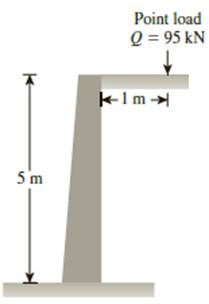Chapter 13, Problem 13.5PPrinciples of Geotechnical Enginee...

9th Edition
Braja M. Das + 1 other
ISBN: 9781305970939

Solutions

Chapter
SectionPrinciples of Geotechnical Enginee...

9th Edition
Braja M. Das + 1 other
ISBN: 9781305970939
Textbook Problem

A 5-m high unyielding retaining wall is subjected to a surcharge point load, Q = 95 kN, on the ground surface (Figure 13.40a). Determine the increase in lateral pressure, σ ′ h , on the wall (on the plane of the section shown) at z = 1, 2, 3, 4, and 5 m due to the point load. (Note: z = distance measured downwards from the top of the wall.)(a)Figure 13.40

To determine

Find the increase in lateral earth pressure σh on the wall when the distance (z) measured downwards from the top of the wall.

Explanation

Given information:

The surcharge point load (Q) is 95 kN.

The distance (x) of load acting from the retaining wall is 1 m.

The height (H) of the retaining wall is 5 m.

The distance measured from top of the retaining wall is 1, 2, 3, 4, and 5 m.

Calculation:

Determine the value of m using the formula.

m=xH

Substitute 1 m for x and 5 m for H.

m=15=0.2

The calculated value of m is less than 0.4, therefore, use Equation (13.19) to calculate the lateral earth pressure.

Determine the value of n when distance measured from top of the retaining wall is 1 m using the relation.

n=zH

Substitute 1 m for z and 5 m for H.

n=15=0.2

Similarly, calculate and tabulate the value of n for the remaining distance as in Table (1).

Determine the lateral earth pressure when the distance measured from top of the retaining wall is 0 m using the formula.

σh=0.28QH2n2(0

Still sussing out bartleby?

Check out a sample textbook solution.

See a sample solution

The Solution to Your Study Problems

Bartleby provides explanations to thousands of textbook problems written by our experts, many with advanced degrees!

Get Started

What determines whether a conventional transmission or a transaxle is used?

Automotive Technology: A Systems Approach (MindTap Course List)

What is Hadoop, and what are its basic components?

Database Systems: Design, Implementation, & Management

In a network, what is the major difference between a server and a client?

Enhanced Discovering Computers 2017 (Shelly Cashman Series) (MindTap Course List)